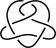# Fibered knot

Updated onIn knot theory, a branch of mathematics, a knot or link K in the 3-dimensional sphere S 3 is called fibered or fibred (sometimes Neuwirth knot in older texts, after Lee Neuwirth) if there is a 1-parameter family F t of Seifert surfaces for K , where the parameter t runs through the points of the unit circle S 1 , such that if s is not equal to t then the intersection of F s and F t is exactly K .

For example:

• The unknot, trefoil knot, and figure-eight knot are fibered knots.
• Fibered knots and links arise naturally, but not exclusively, in complex algebraic geometry. For instance, each singular point of a complex plane curve can be described topologically as the cone on a fibered knot or link called the link of the singularity. The trefoil knot is the link of the cusp singularity z 2 + w 3 ; the Hopf link (oriented correctly) is the link of the node singularity z 2 + w 2 . In these cases, the family of Seifert surfaces is an aspect of the Milnor fibration of the singularity.

A knot is fibered if and only if it is the binding of some open book decomposition of S 3 .

## Knots that are not fibered

The Alexander polynomial of a fibered knot is monic, i.e. the coefficients of the highest and lowest powers of t are plus or minus 1. Examples of knots with nonmonic Alexander polynomials abound, for example the twist knots have Alexander polynomials qt − (2q + 1) + qt−1, where q is the number of half-twists. In particular the Stevedore's knot is not fibered.

## References

Similar Topics
Umrao Jaan (1981 film)
Gianni Bonagura
Kōhei Murakami
Topics